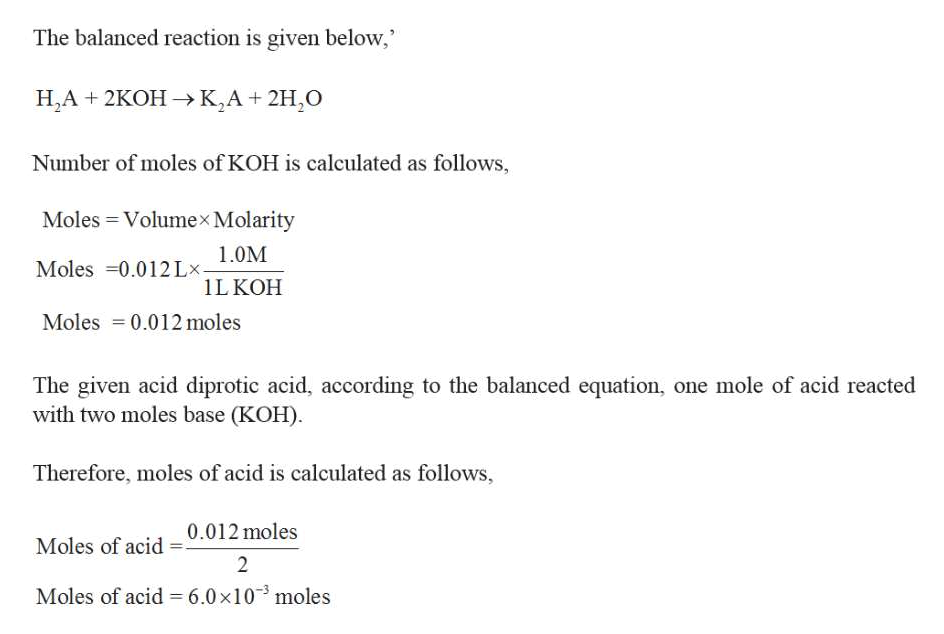# A 4.50−g quantity of a diprotic acid was dissolved in water and made up to exactly 250 mL. Calculate the molar mass of the acid if 25.0 mL of this solution required 12.0 mL of 1.00 M KOH for neutralization. Assume that both protons of the acid were titrated.

Question
20 views

A 4.50−g quantity of a diprotic acid was dissolved in water and made up to exactly 250 mL. Calculate the molar mass of the acid if 25.0 mL of this solution required 12.0 mL of 1.00 M KOH for neutralization. Assume that both protons of the acid were titrated.

check_circle

Step 1

Given,

Diprotic acid = 4.50 g in 250 mL

...help_outlineImage TranscriptioncloseThe balanced reaction is given below, H,A 2KOHK,A 2H2O Number of moles of KOH is calculated as follows, Moles Volumex Molarity 1.0M Moles 0.012Lx 1LKOH Moles 0.012 moles The given acid diprotic acid, according to the balanced equation, one mole of acid reacted with two moles base (KOH) Therefore, moles of acid is calculated as follows, 0.012moles Moles of acid 2 Moles of acid = 6.0x103 moles fullscreen

### Want to see the full answer?

See Solution

#### Want to see this answer and more?

Solutions are written by subject experts who are available 24/7. Questions are typically answered within 1 hour.*

See Solution
*Response times may vary by subject and question.
Tagged in

### General Chemistry